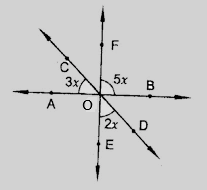"
">

# In the figure, find the value of $x$."

Given:

$\angle BOF=5x, \angle AOC=3x, \angle DOE=2x$

To do:

We have to find the value of $x$.

Solution:

We know that,

Vertically opposite angles are equal.

Sum of the angles on a straight line is $180^o$.

Therefore,

$\angle DOB=\angle AOC=3x$       (Vertically opposite angles)

$FOE$ is a straight line.

This implies,

$\angle BOF+\angle BOD+\angle DOE = 180^o$

$5x + 3x + 2x = 180^o$

$10x= 180^o$

$x=\frac{180^o}{10}$

$x=18^o$

Hence, $x = 18^o$.

Updated on: 10-Oct-2022

28 Views